# Cylinder

Let’s have a look at the famous historical tower of Italy!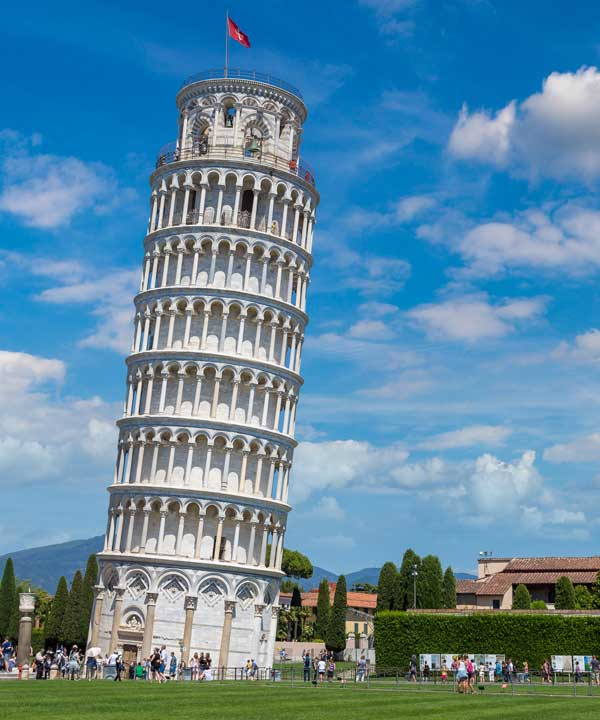Yes, it's the Leaning Tower of Pisa, which is cylindrical in shape.

In the late 14th century, the word "cylinder" is derived from Latin (Cylindrus) and Greek (Kylindros) words, which mean "roll",  "roller", and "tumblr".

In this chapter, we are going to look at the cylinder formula, cylinder volume, cylinder surface area. Try your hands at cylinder surface area calculator and interactive questions for better understanding.

But before going into those details about cylinder, let’s look at the definition of cylinder and shape of cylinder by exploring the simulation, given in the chapter.

## What is a Cylinder?

Cylinder definition: A cylinder shape in mathematics is a three-dimensional solid figure which consists of two circular bases connected with two parallel lines. A cylinder surface area calculator and other formulae help in calculating the various properties of cylinders.

These two parallel lines form a curved surface that is bounded by two circles at the top and bottom.

## Shape of a Cylinder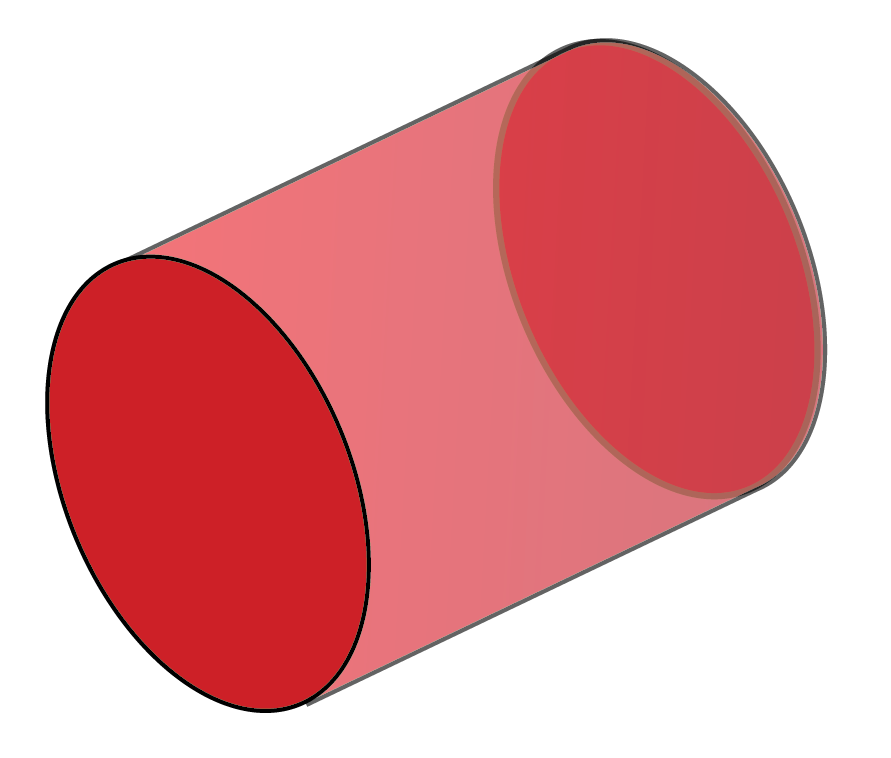Have you observed this shape in your everyday life?

Let us look at a few objects that are in a cylinder shape.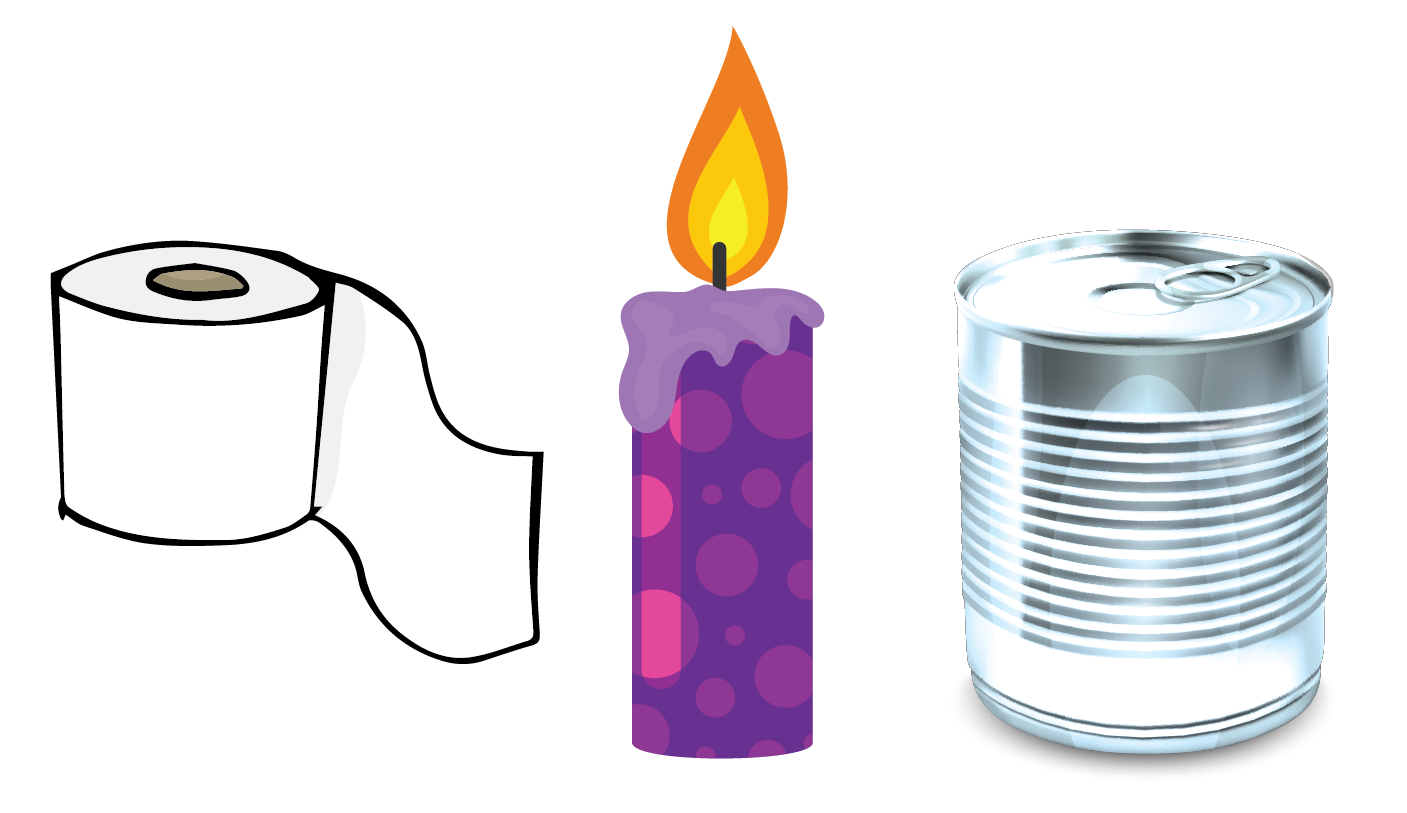### Types of Cylinder

There are four different types of cylinders. They are:

• Right circular cylinder
• Oblique cylinder
• Elliptic cylinder
• Right circular hollow cylinder or cylindrical shell

### Right Circular Cylinder

If the axis of the two parallel lines is perpendicular to the center of the base, it is called the right circular cylinder.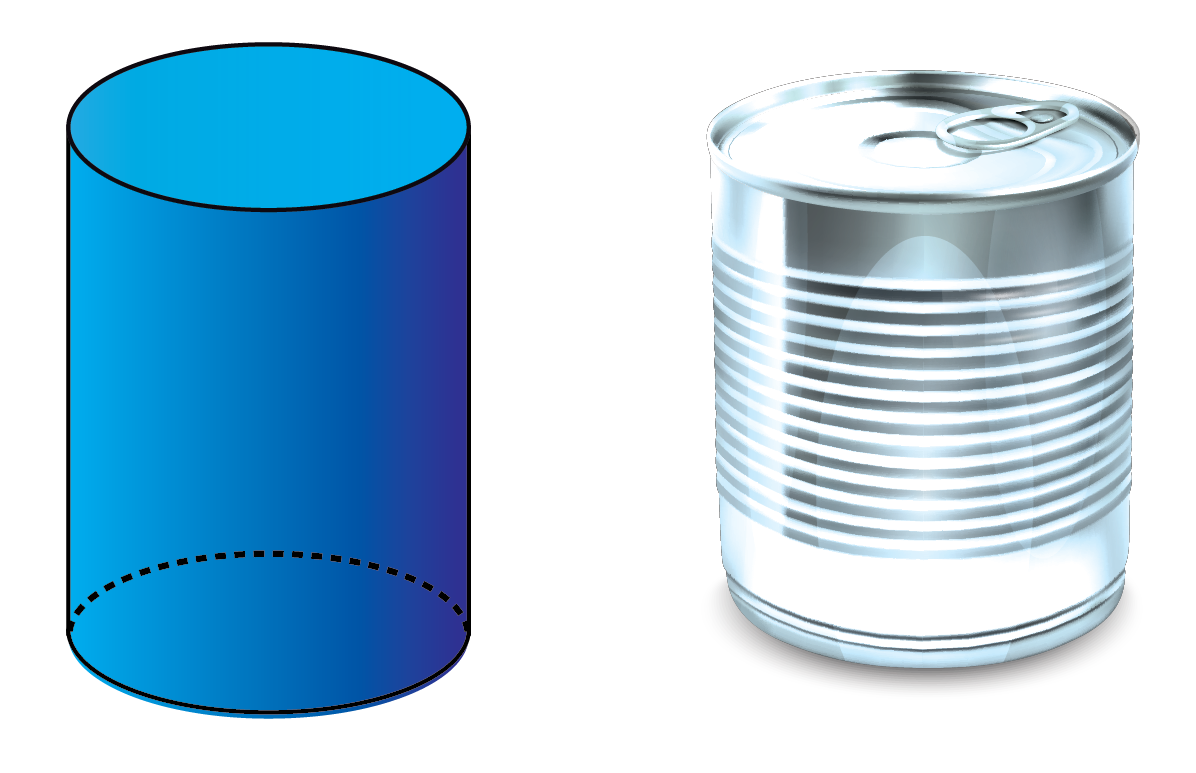### Oblique Cylinder

An oblique cylinder is one whose sides lean over the base.

In an oblique cylinder, the sides are not perpendicular to the center of the base.

You remember the Leaning Tower of Pisa discussed above, right?

The Leaning Tower of Pisa is the best example of an oblique cylinder.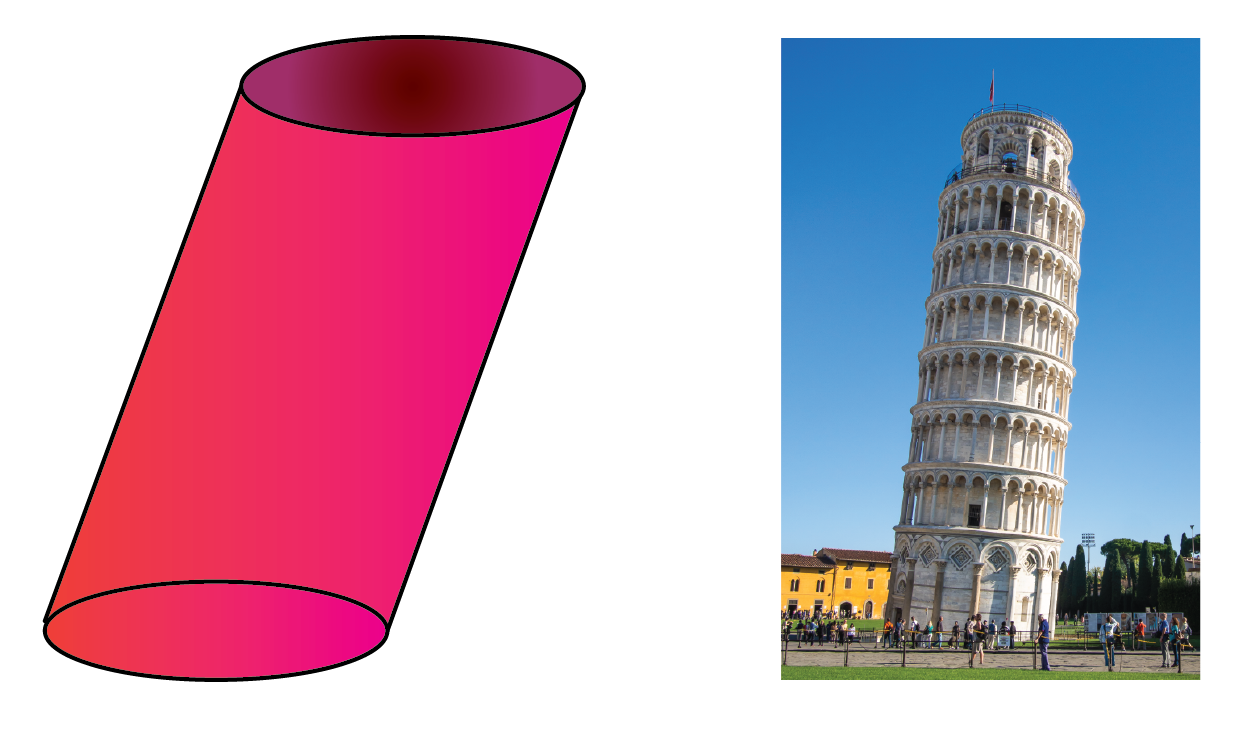### Elliptic Cylinder

A cylinder whose base is in the form of an ellipse is called an elliptic cylinder.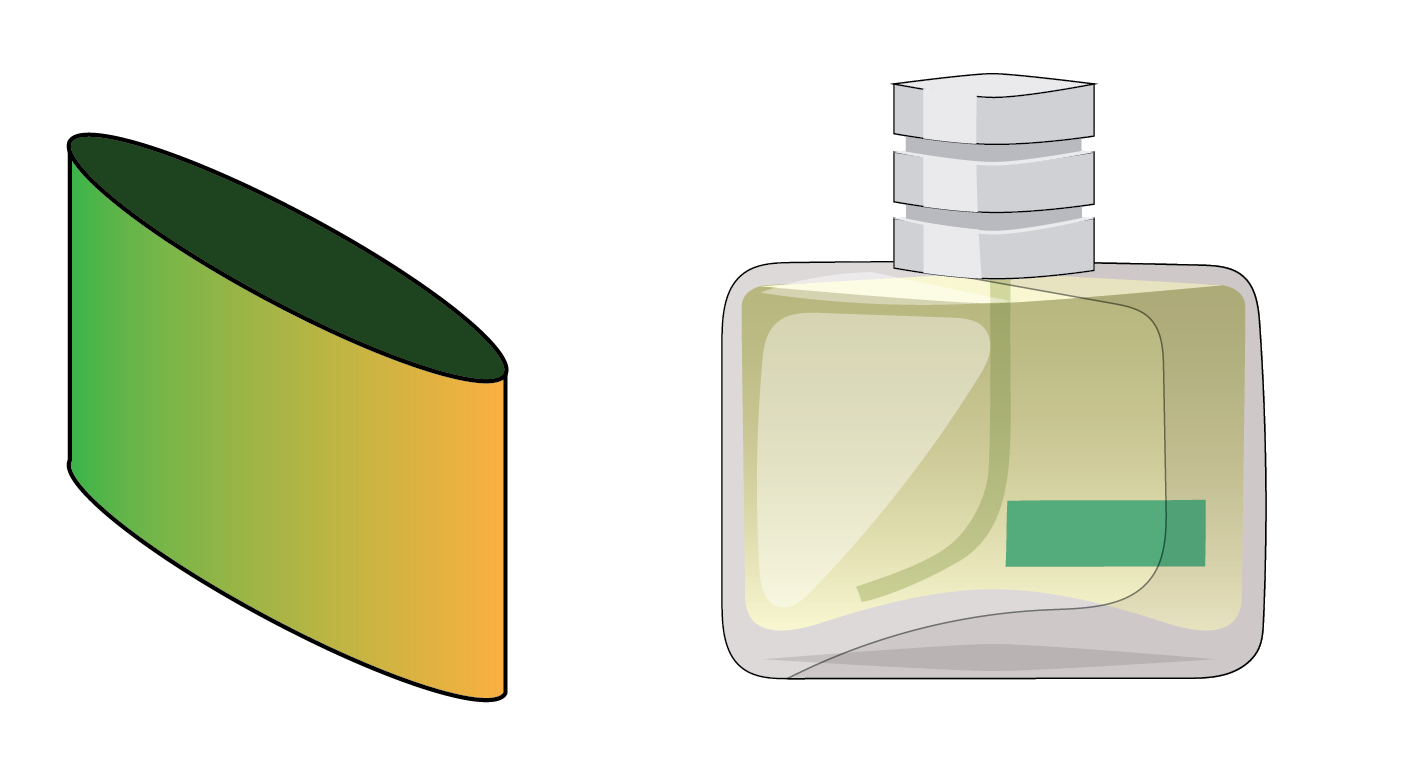The below simulation will help you to know the different types of cylinders.

Drag and spin the cylinder to see the right circular, elliptic, and oblique cylinders.

### Right Circular Hollow Cylinder (Cylindrical Shell)

The right circular hollow cylinder also called a "cylindrical shell", consists of two right circular cylinders bounded one inside the other.

The point of the axis is common and is perpendicular to the central base.

It is different from the right circular cylinder since it is hollow in nature, i.e. there is some space or void present inside.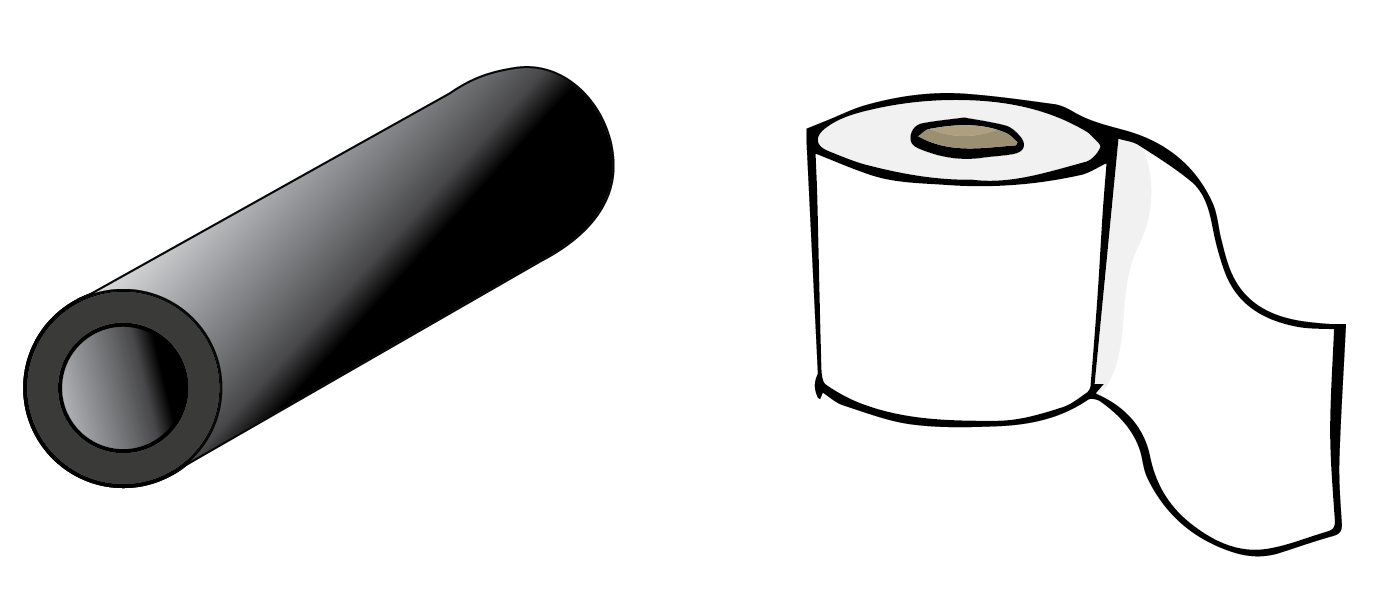The simulation below will let you know the inner and outer surface area of the hollow circular cylinder.

## Formulae of a Cylinder

### Right Circular Cylinder: Total Surface Area (TSA)

The total surface area of a cylinder defines the total area that it occupies.

If we observe the formation of a cylinder, we can see two circles and one curved sheet.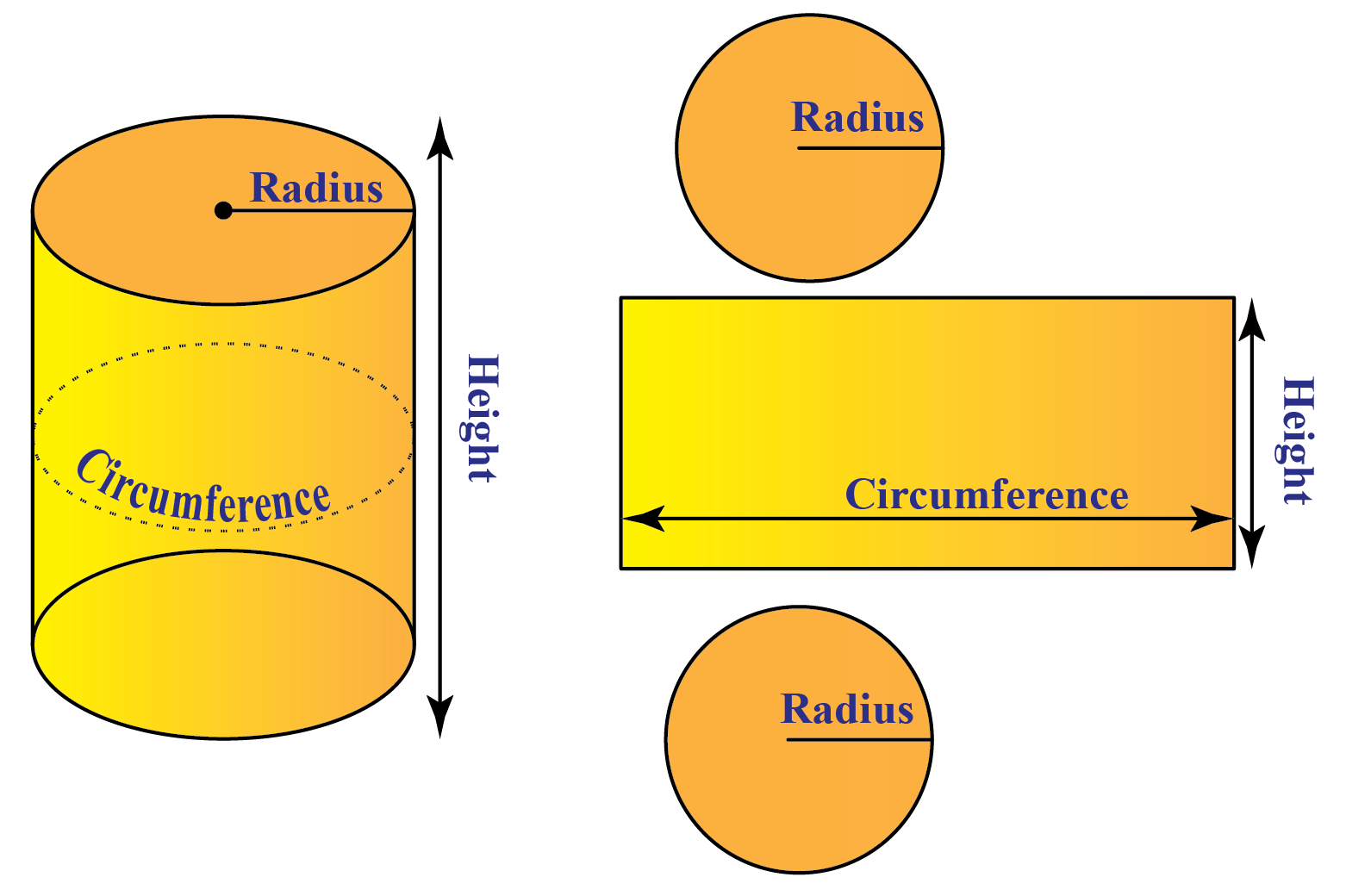So, in order to find out the total surface area of a cylinder, we calculate the curved surface area and the area of two circles.

Curved Surface Area(CSA) = Circumference x Height

CSA= 2𝛑r x h

Area of a Circle = 𝛑r2

Total Surface Area (TSA)= Curved Surface Area + 2(Area of a circle)

Note: There are two circles, so we multiply by 2

TSA= 2𝛑rh + 𝛑r2

 TSA of a Right Circular Cylinder = 2𝛑r(h+r)

### Right Circular Cylinder: Volume

The cylinder volume defines the density or amount of space it occupies.

The volume of a cylinder = Area of a circle x height

Volume = 𝛑r2 x h

 The volume of a Right Circular Cylinder = 𝛑r2h

### Oblique Cylinder: Total Surface Area (TSA)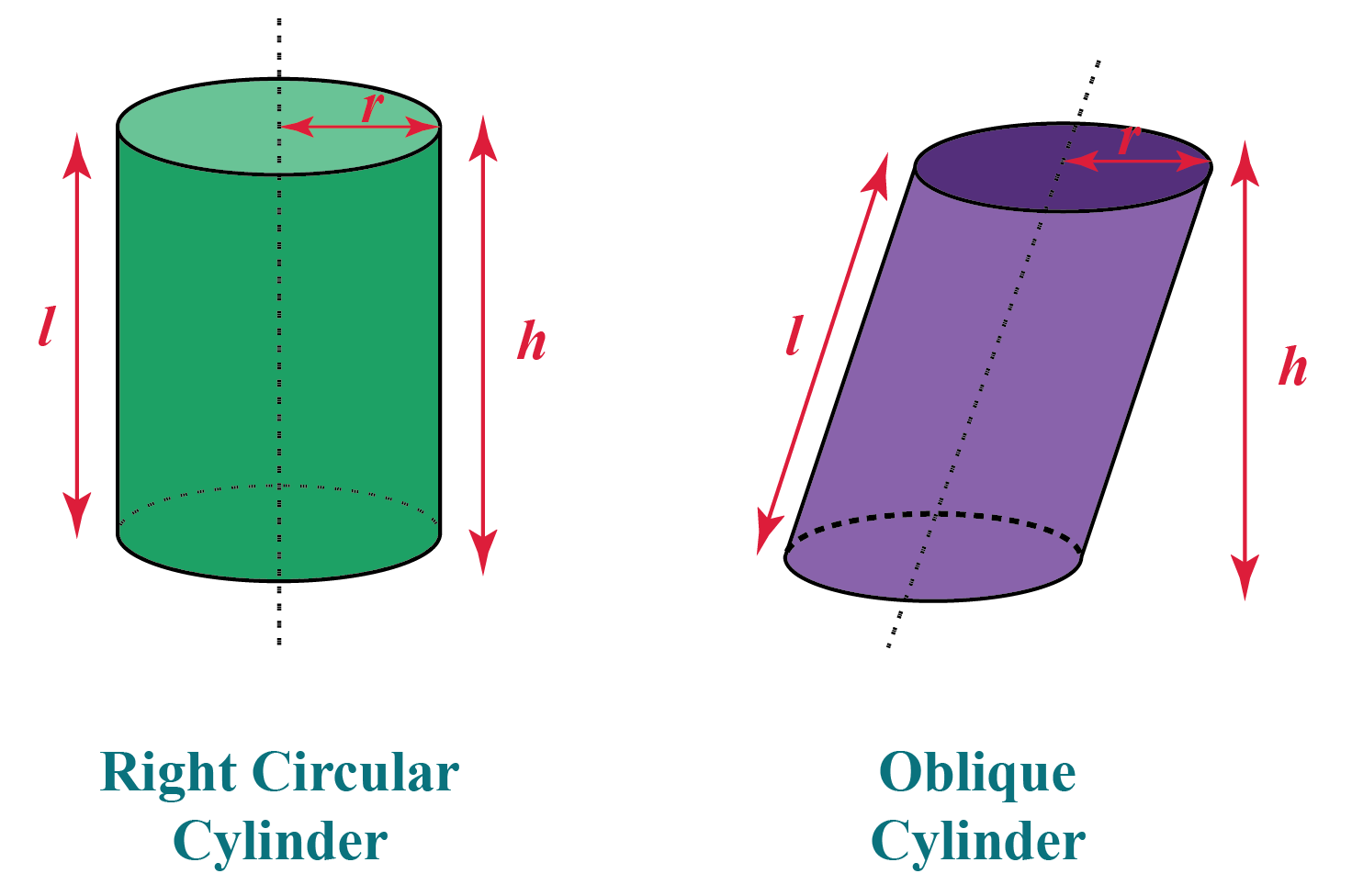In a right circular cylinder, the length will coincide with the height and the length of the central axis.

But, in the case of an oblique cylinder, the length will coincide only with the central axis but not with the vertical height.

Total surface area of a right circular cylinder = 2𝛑r(h+r)

To calculate the total surface area of an oblique cylinder, we use slanting length (l) instead of height (h).

 TSA of Oblique Cylinder = 2𝛑r(l+r)

### Oblique Cylinder: Volume

In general, the volume of an object is the base area along with its height.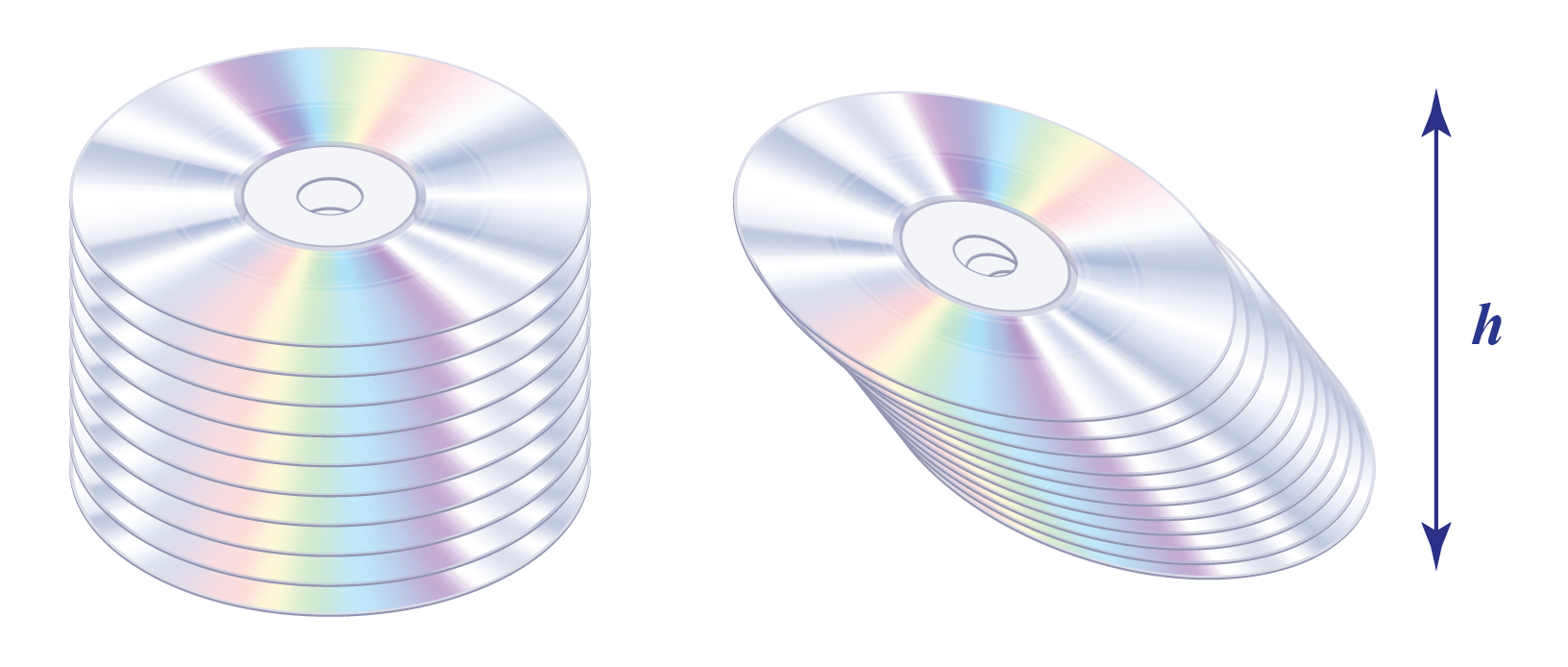The heights and area of these two cylinders are the same.

Therefore the volume of an oblique cylinder is the same as the volume of a right circular cylinder.

 Volume of Oblique Cylinder = 𝛑r2h

### Elliptic Cylinder: Total Surface Area (TSA)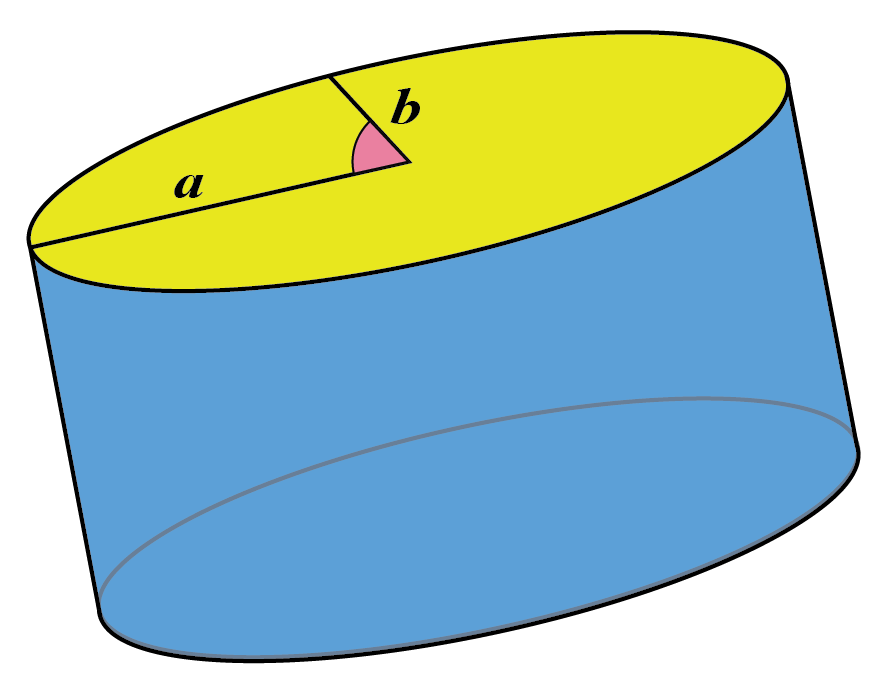a = Semi-major axis

b = Semi-minor axis.

We derive the total surface area from the total surface of a right circular cylinder.

 TSA of elliptic cylinder = π(a+b)h+2πab

### Elliptic Cylinder: Volume

The base of an elliptic cylinder is in the form of an ellipse.

So, there are two radii a and b.

 The volume of elliptic cylinder = πabh

### Cylindrical Shell: Total Surface Area (TSA)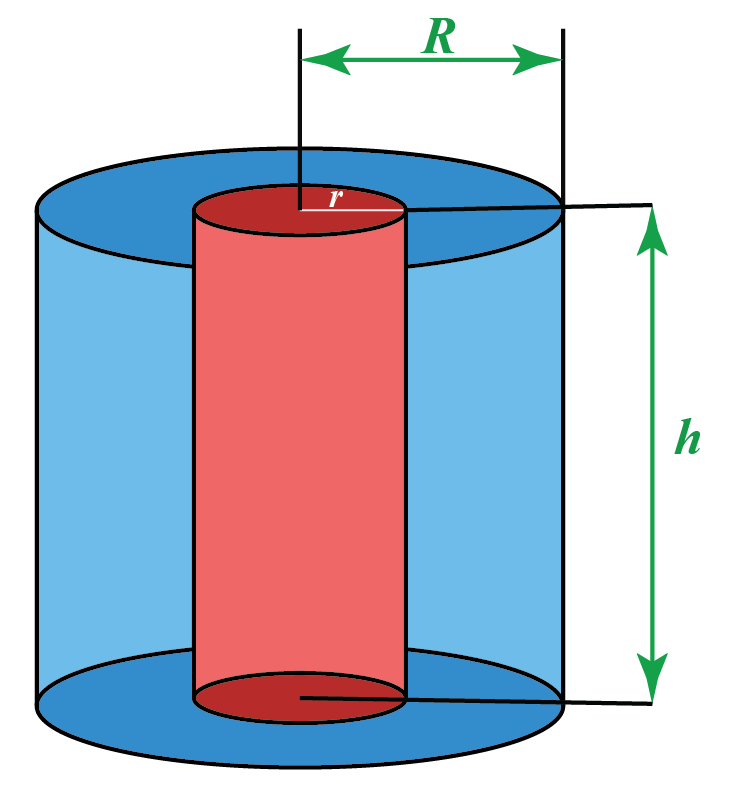The hollow cylinder or cylindrical shell has an inner radius and outer radius.

Therefore,

 TSA of Cylindrical Shell = 2π(R+r)h+2π(R2–r2)

### Cylindrical Shell: Volume

We know that volume of a cylinder = 𝛑r2h

Here, in a cylindrical shell, we have two radii "R" and "r".

Therefore,

 The volume of Cylindrical Shell = π(R2–r2)h

## Properties of a Cylinder

There are a few properties that define a cylinder.

•   By cylinder definition, it has two curved edges, one curved surface, and two flat faces.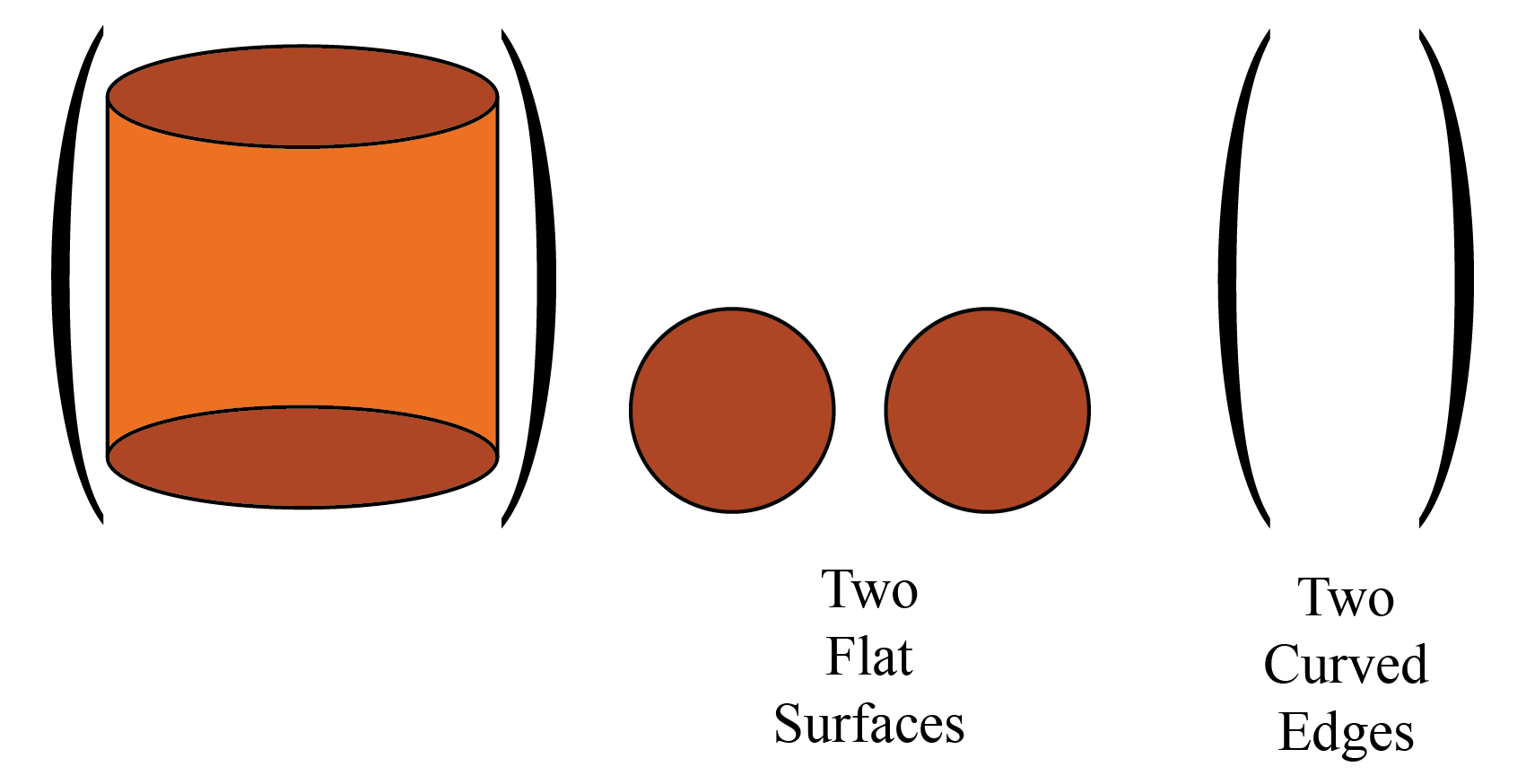• Unlike a cone, cube, or cuboid, a cylinder does not have any vertex. It means there is no specific corner present in the cylinder.
• The base and the top of the cylinder are identical, i.e they have the same base  either circular or elliptical.

## Solved Examples

 Example 1

Anna has a beautiful candleholder, and she goes to the shop to buy a candle.

Help her figure out the right shape of the candle which can fit in her candleholder.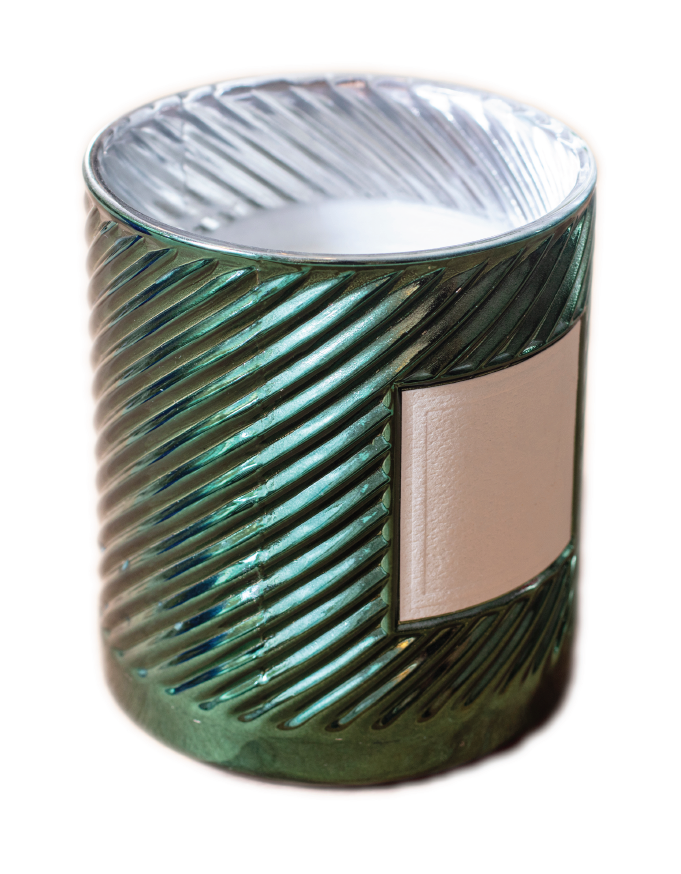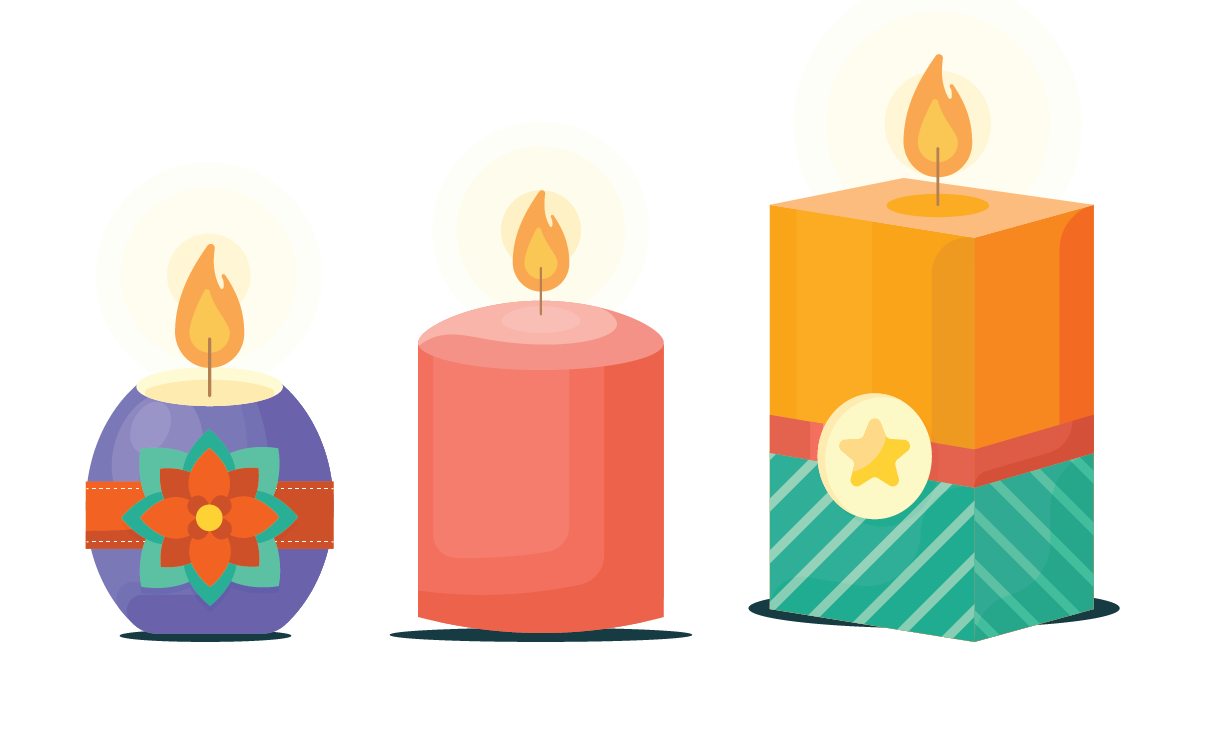Solution

The first candle resembles a cone shape.

The second candle resembles a cylinder.

The third candle resembles a cuboid.

The candleholder is in the form of a cylinder.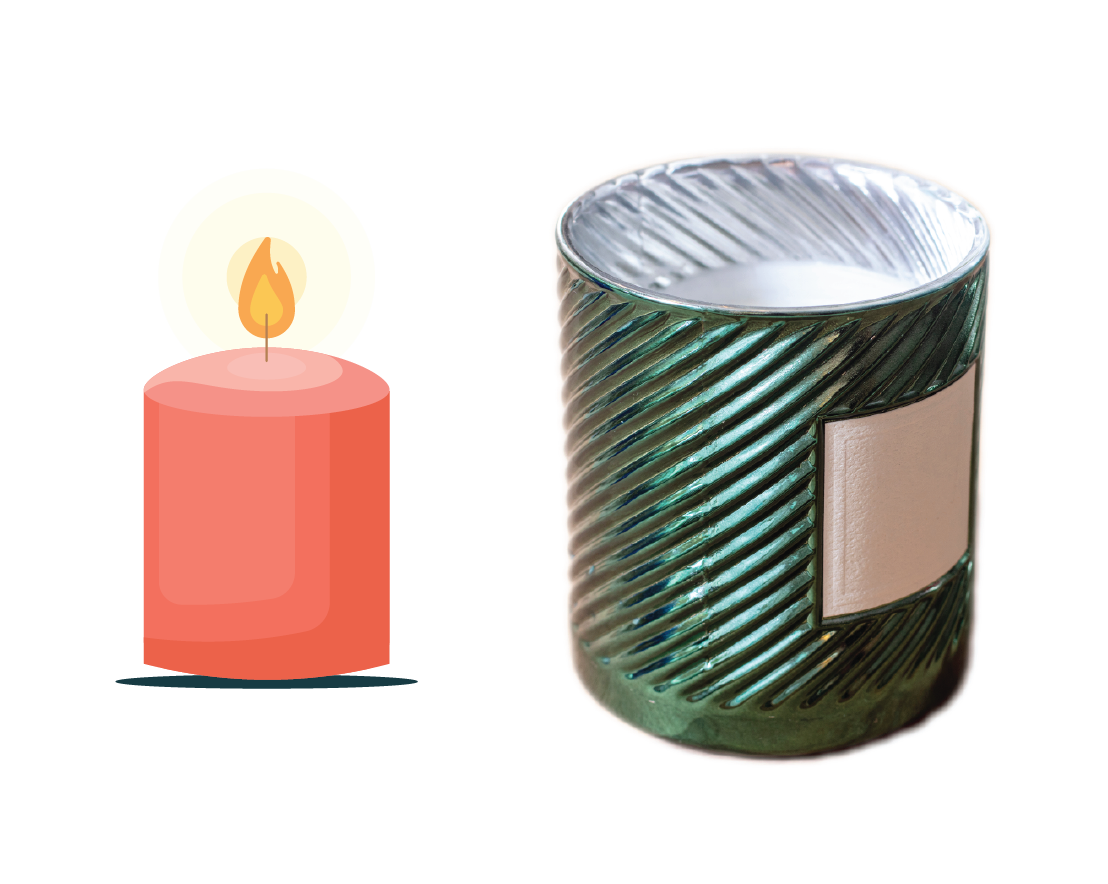Cylinder shape candle fits into the candleholder.

 $$\therefore$$ Cylinder shape candle is the right choice.
 Example 2

James calls a plumber to fix the water leakage problem in a pipe.

The plumber asks James to buy a new pipe to replace the old one.

Which is the suitable shape?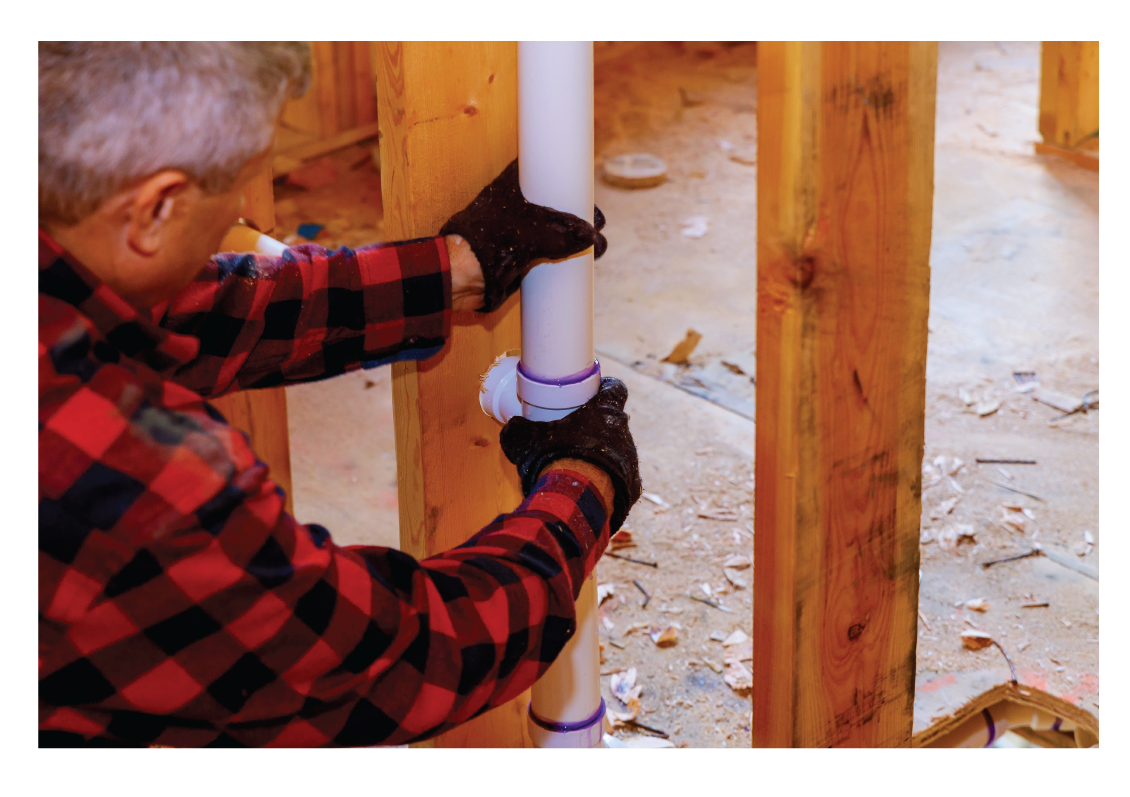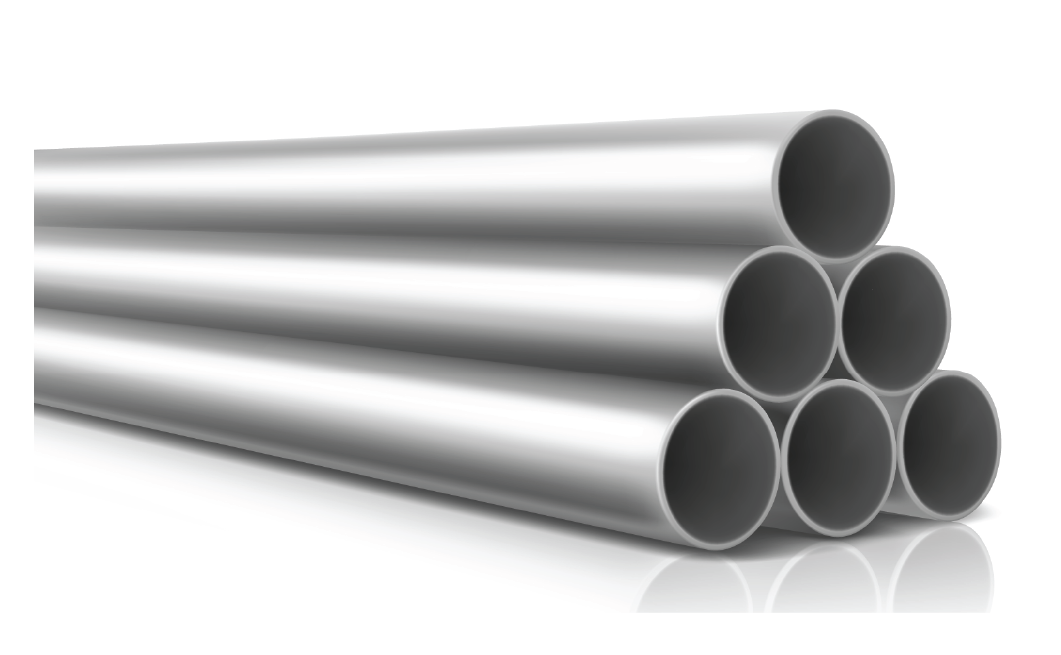Hollow pipes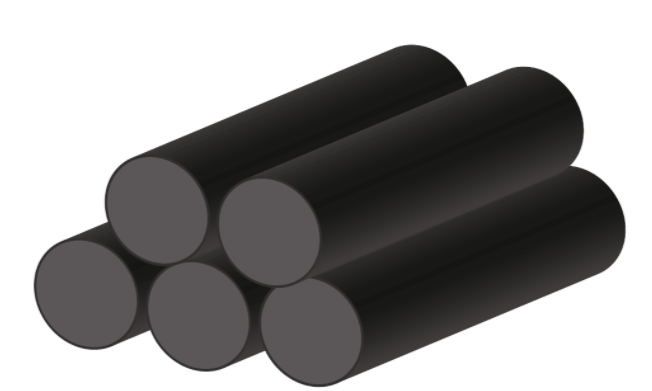Closed-end pipes

Solution

The first image shows closed-end pipes.

These closed-end pipes are in the form of a right circular cylinder.

These closed-end pipes do not allow the flow of water.

The hollow pipes resemble cylindrical shells.

A hollow pipe is suitable here since it will allow the flow of water.

 $$\therefore$$ Hollow Pipe is a suitable pipe.
 Example 3

Britto wants to buy a can that can hold 1 gallon of oil.

The radius of the can is 5 inches.

Help Britto find the height of the can she has to buy.

Hint: The can is in the form of a cylinder.

Solution

Volume V= 1 gallon

1 gallon= 231 cubic inches

The volume of a cylinder V = 𝛑r2h

\begin{equation}231=\frac{22}{7} \times(5)^{2} \times h\end{equation}

\begin{equation}\frac{231 \times 7}{22 \times 25}=h\end{equation}

\begin{equation}h=2.94\end{equation}

Therefore, the height of the can should be 2.94 inches.

 $$\therefore$$ Height = 2.94 inches.
 Example 4

Emma has an old cylindrical water tank at her home.

The radius is 40 inches and the height is 150 inches.

She wants to replace it with a new one with the same dimensions.

Help Emma figure out the area of the water tank.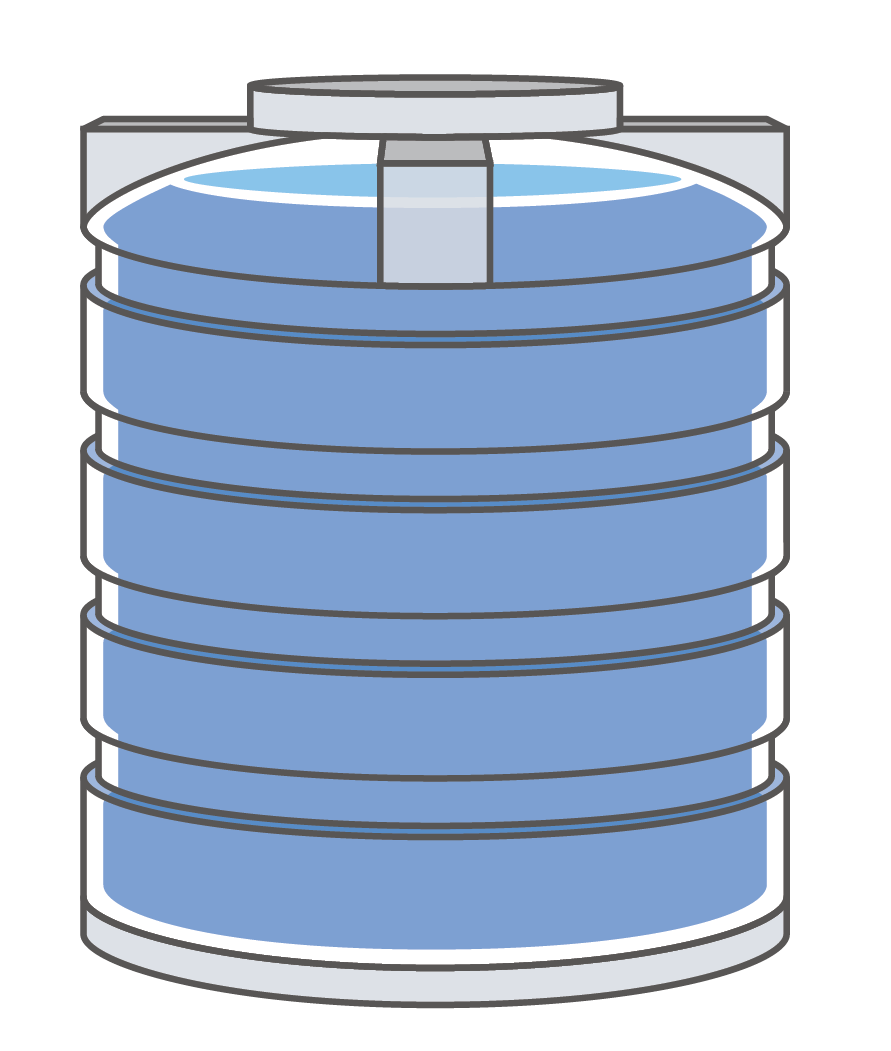Solution

The water tank is in the form of a cylinder.

Total Surface Area of a cylinder =  2𝛑r(h+r)

\begin{align}\text{TSA}&=2 \times \frac{22}{7} \times 40(150+40)\\ \text{TSA}&=2 \times \frac{22}{7} \times 7600\\ \text{TSA} &= 47,771.42 sq.inches\end{align}

 $$\therefore$$ Area of the water tank = 47,771.42 sq.inches
 Example 5

Elsa goes to a shop to buy a cylindrical pencil box.

The outside of the pencil box is made of fiber with a radius of 4 inches and the inside is made of sponge with a radius of 2 inches.

The height of the pencil box is given as 20 inches.

Help Elsa figure out the total surface area of the pencil box.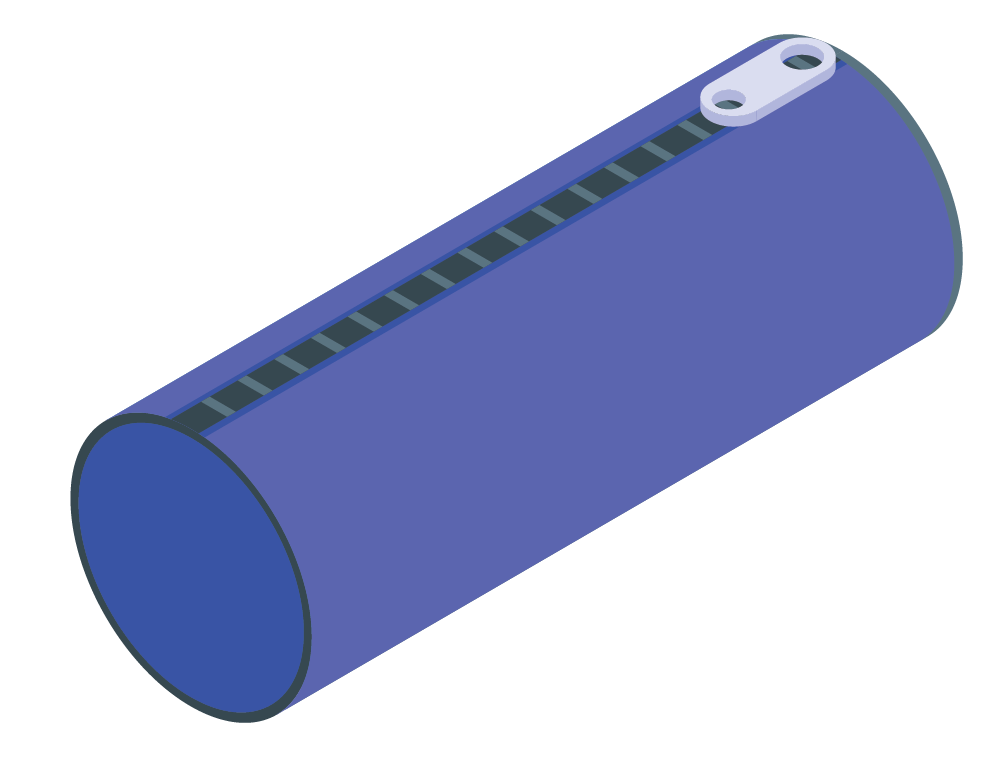Solution

Outer radius R = 4 inches

Inner radius r = 2 inches

Height h = 20 inches

This pencil box resembles a cylindrical shell.

Therefore,                                                                                                                            TSA of the cylindrical shell = 2π(R+r)h+2π(R2–r2)

\begin{align}\text{TSA}&=2 \times \frac{22}{7} \times (4+2)+2 \times \frac{22}{7} \times (16-4)\\ \text{TSA}&=2 \times \frac{22}{7} \times (6)+2 \times \frac{22}{7} \times(12)\\ \text{TSA}&= \frac{264}{7}+ \frac{528}{7}\\ \text{TSA}&=113.14 sq.inches\end{align}

 $$\therefore$$ TSA of a pencil box = 113.14 sq.inchesThink Tank

What is the relationship between a prism and a cylinder?

## Interactive Questions

Here are a few activities for you to practice. Select/Type your answer and click the "Check Answer" button to see the result.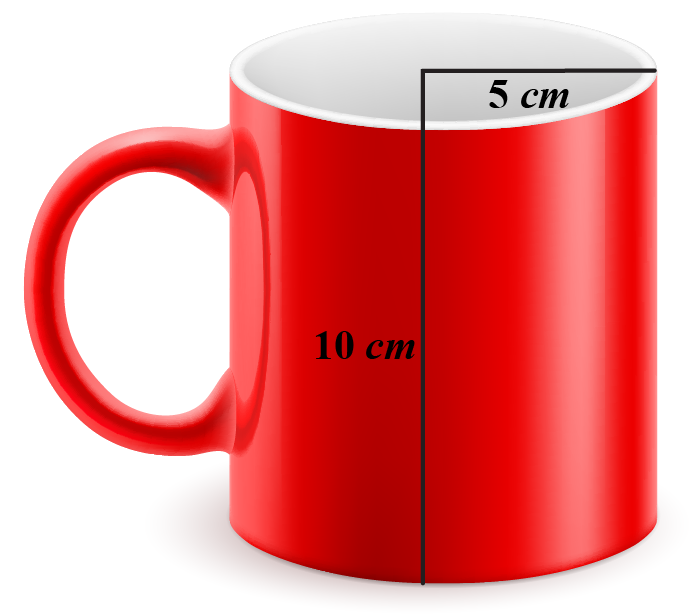## Let's Summarize

We hope you enjoyed learning about the Cylinder with simulations and practice questions. Now you will be able to easily solve problems on the cylinder formula, cylinder volume, cylinder definition, cylinder Shape, and cylinder surface area calculator.

At Cuemath, our team of math experts is dedicated to making learning fun for our favorite readers, the students!

Through an interactive and engaging learning-teaching-learning approach, the teachers explore all angles of a topic.

Be it worksheets, online classes, doubt sessions, or any other form of relation, it’s the logical thinking and smart learning approach that we, at Cuemath, believe in.

## 1. What is a cylinder shape?

A cylinder is a three-dimensional solid figure which consists of two circular bases connected with two parallel lines.

These two parallel lines form a curved surface which is bounded by two circles at the top and the bottom.

## 2. How many edges does a cylinder have?

A cylinder has two curved edges.

## 3. How many sides does a cylinder have?

There are two flat circular faces and one curved surface area present in a cylinder.

In a cylinder, we do not use the word "sides" since the cylinder has a curved surface.More Important Topics
Numbers
Algebra
Geometry
Measurement
Money
Data
Trigonometry
Calculus